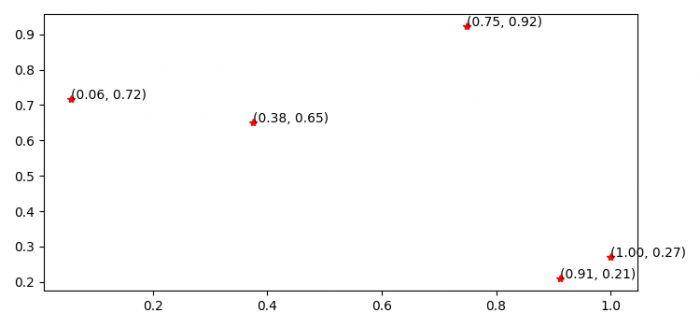# Showing points coordinate in a plot in Python Matplotlib

To show points coordinate in a plot in Python, we can take the following steps −

## Steps

• Set the figure size and adjust the padding between and around the subplots.

• Initilize a variable N and create x and y data points using numpy.

• Zip the x and y data points; iterate them and place coordinates.

• To display the figure, use show() method.

## Example

import matplotlib.pyplot as plt
import numpy as np

plt.rcParams["figure.figsize"] = [7.50, 3.50]
plt.rcParams["figure.autolayout"] = True

N = 5

x = np.random.rand(N)
y = np.random.rand(N)

plt.plot(x, y, 'r*')

for xy in zip(x, y):
plt.annotate('(%.2f, %.2f)' % xy, xy=xy)

plt.show()

## Output

It will produce the following output −